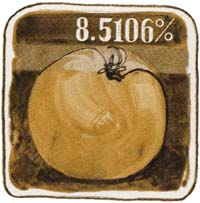Section 6: Functions84
Notice that the calculated answer writes over the percent rate in the X-register, and the base number is preserved in the Y-register.
Since the total tomato weight is still in the Y-register and the weight of the water in the tomato is in the X-register, the weight of the remainder can be obtained by simply subtracting:
Keystrokes Display

 470
The weight of the water.
–
 30
The gram weight of solid matter in the 500-gram tomato.
Percent of Change
The %CH (percent of change) function is a two-number function that calculates the percent increase or decrease from a number in the Y-register to the number in the X-register. To find the precent of change:
 1. Key in the base number (usually, the number that happens first in time). 2. Press ENTER  . 3. Key in the second number. 3. Execute the %CH function form the display. Percent of change is calculated as %CH = [(x – y) 100] ÷ y. (When y = 0 a value of 9.999999999 × 1099 is placed in the X-register and the calculator displays OUT OF RANGE.)
 Example: Tomato grower Flem Snopes has found that he can decrease the amount of water in the tomatoes he is growing. His typical tomato weighs about 500 grams. He has found that only 430 grams of the total weight is water, compared to 470 grams of water in the tomato from the previous example. What is the percent of change of water amount between the average tomato and Snopes’ tomato?